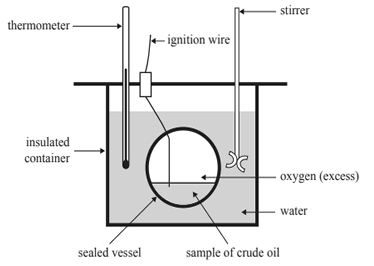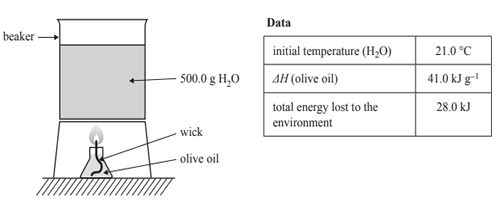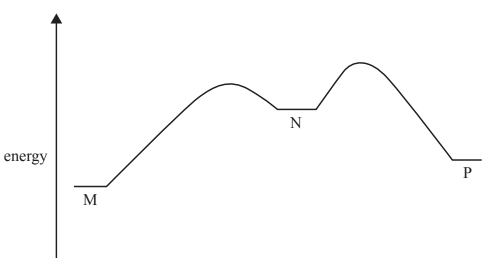Thermochemistry (2017 VCE) What is the total energy released, in kilojoules, when 100 g of butane and 200 g of octane undergo combustion in the presence of excess oxygen? A. 9760 B. 14 600 C. 17 300 D. 19 500 Solution2) The heat of combustion of a sample of crude oil is to be determined using a bomb calorimeter. All of the students in a class are given the same method to follow. The apparatus used by the students is shown below.For this experiment, the students could maximise A. precision by using a digital thermometer ± 0.2 °C. B. validity by calculating the heat of combustion per mole. C. accuracy by taking samples from three different sources. D. uncertainty by having all students closely follow the same experimental procedure Solution3) A sample of olive oil with a wick in a jar is ignited and used to heat a beaker containing 500.0 g of water, H2O. The relevant data for the experiment is included in the table below.After complete combustion of 2.97 g of olive oil, the final temperature of the water, in degrees Celsius, would be A. 44.9 B. 58.0 C. 65.9 D. 79.3 Solution4) The following energy profile shows the results obtained during an enzyme-catalysed reaction. Each stage of the reaction is labelled: M represents the initial reactants, N represents a stable intermediate and P represents the final products.Which one of the following statements is correct? A. The energy change from M to N is exothermic and the energy change from N to P is exothermic. B. The energy change from M to P is exothermic and the energy change from N to P is endothermic. C. The energy change from M to N is endothermic and the energy change from N to P is endothermic. D. The energy change from M to N is endothermic and the energy change from M to P is endothermic. Solution